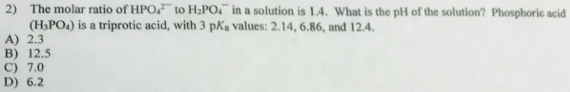# Problem: The molar ratio of HPO42- to H2PO4- in a solution is 1.4. What is the pH of the solution? Phosphoric acid (H3PO4) is a triprotic acid, with 3 pKa values: 2.14, 6.86, and 12.4.A. 2.3B. 12.5C. 7.0D. 6.2

###### FREE Expert Solution

Following the Henderson-Hasselbach equation, pH = pKa + log ([base]/[acid])

There are 3 pKa values available but only one should be used.

The lowest pKa is for the proton coming from H3PO4. The second pKa is for the proton that will be dissociated from H2PO4-. And the last will be for the proton from HPO42-.###### Problem Details

The molar ratio of HPO42- to H2PO4- in a solution is 1.4. What is the pH of the solution? Phosphoric acid (H3PO4) is a triprotic acid, with 3 pKa values: 2.14, 6.86, and 12.4.

A. 2.3

B. 12.5

C. 7.0

D. 6.2